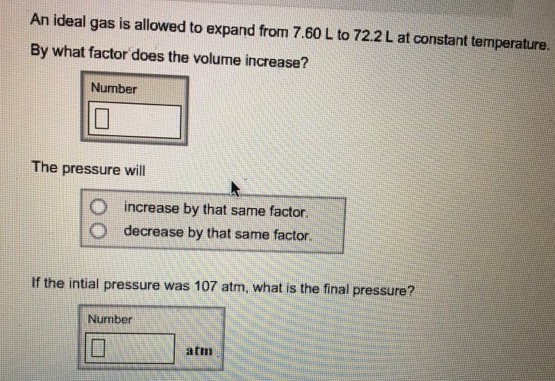# Problem: An ideal gas is allowed to expand from 7.60 L to 72.2 L at constant temperature. By what factor does the volume increase? The pressure will increase by that same factor. decrease by that same factor. If the initial pressure was 107 atm, what is the final pressure?

###### FREE Expert Solution

According to the Boyle's Law, Volume is inversely proportional to Pressure

88% (38 ratings)###### Problem Details

An ideal gas is allowed to expand from 7.60 L to 72.2 L at constant temperature. By what factor does the volume increase? The pressure will increase by that same factor. decrease by that same factor. If the initial pressure was 107 atm, what is the final pressure?